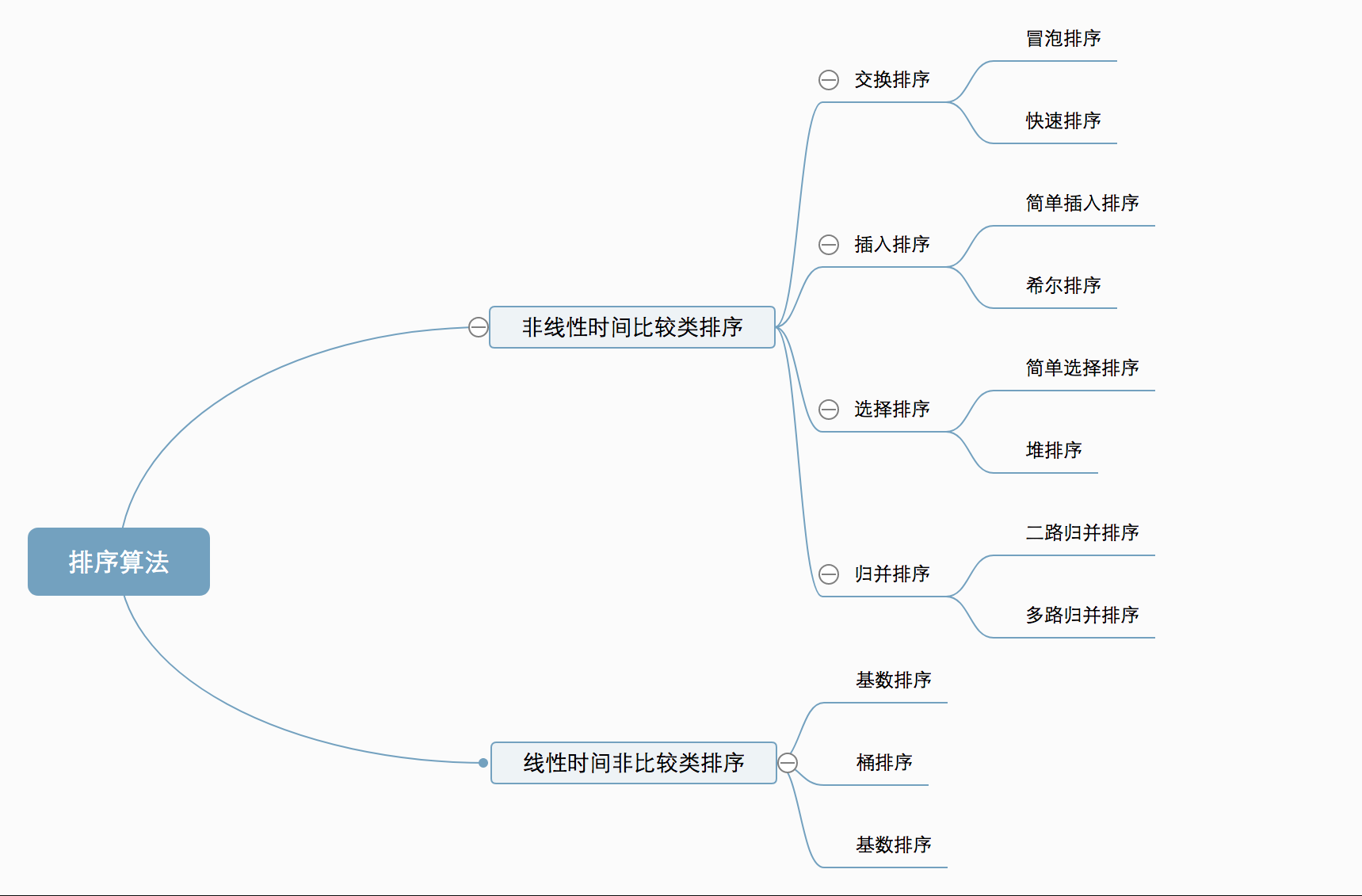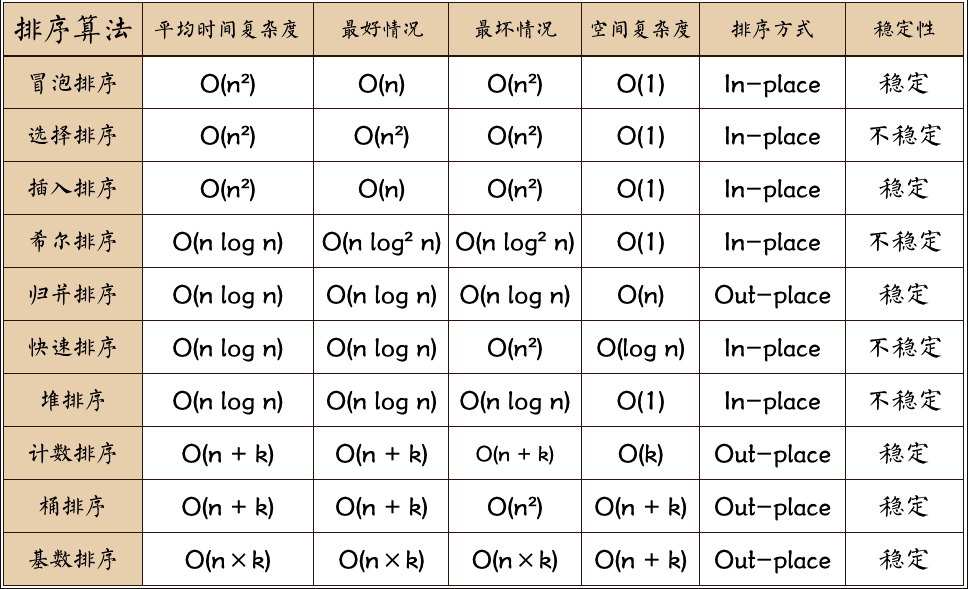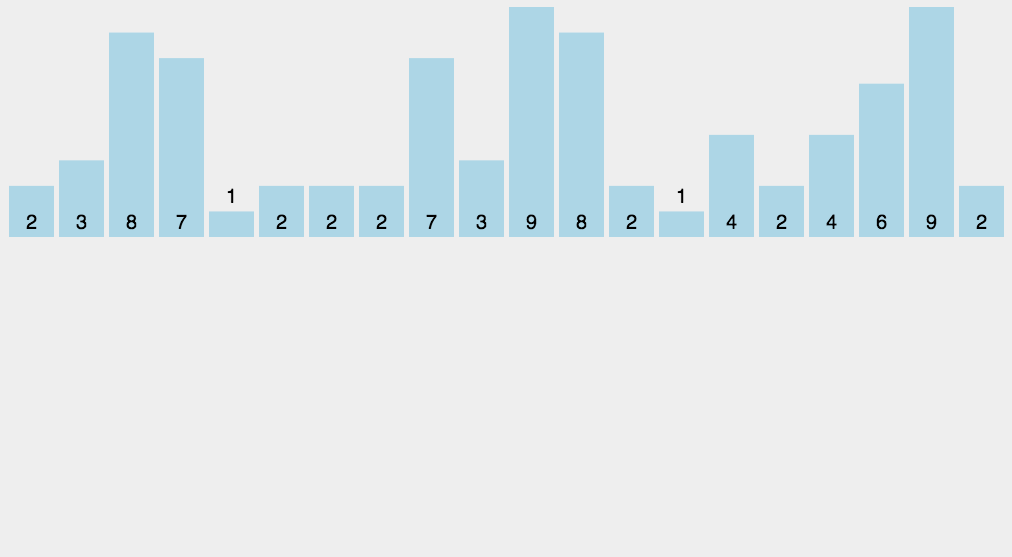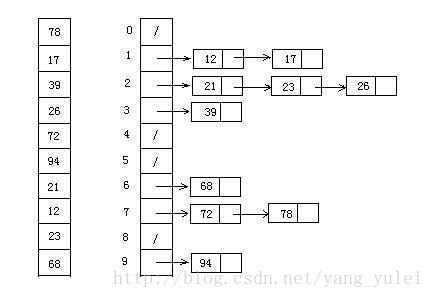#雪月书韵茶香

/

## 常见排序算法总结# 常见排序算法总结

### 1、基本介绍

``````排序算法比较基础，但是设计到很多计算机科学的想法，如下：
``````
``````1. 比较和非比较的策略
``````
``````2. 迭代和递归的实现
``````
``````3. 分而治之思想
``````
``````4. 最佳、最差、平均情况时间复杂度分析
``````
``````5. 随机算法
``````

### 2、排序算法的分类

#### 算法分类#### 算法总结### 3、冒泡排序

#### （1）冒泡排序的介绍

``````冒泡排序是一种简单的排序算法。它重复地走访过要排序的数列，一次比较两个元素，如果它们的顺序错误就把它们交换过来。走访数列的工作是重复地进行直到没有再需要交换，也就是说该数列已经排序完成。这个算法的名字由来是因为越小的元素会经由交换慢慢“浮”到数列的顶端。
``````

#### （2）冒泡排序的原理：

``````1. 如果元素大小关系不正确，交换这两个数（在本例中为a> b），
``````
``````2. 比较一对相邻元素（a，b），
``````
``````3. 重复步骤1和2，直到我们到达数组的末尾（最后一对是第（N-2）和（N-1）项，因为我们的数组从零开始）
``````
``````4. 到目前为止，最大的元素将在最后的位置。 然后我们将N减少1，并重复步骤1，直到N = 1。
``````

#### （3）动图演示#### （4）代码演示

``````    public static void bubbleSort1(int array[]){
//声明临时变量 实现冒泡
int t =0;
for (int i = 0; i <array.length ; i++) {
for (int j = 0; j <array.length-1-i ; j++) {
if (array[j]>array[j+1]){
t = array[j];
array[j] = array[j+1];
array[j+1] = t;
}
}
}
}

public static void selecttionSort2(int[] array){
//不声明临时变量实现冒泡排序
for (int i = 0; i <array.length ; i++) {
for (int j = i+1; j <array.length ; j++) {
if (array[i] > array[j]){
array[j] = array[j]+array[j+1];
array[j+1] = array[j]-array[j+1];
array[j] = array[j] - array[j+1];
}

}
}
}
``````

### 4、选择排序

#### （1）选择排序的介绍

``````选择排序(Selection-sort)是一种简单直观的排序算法。它的工作原理：首先在未排序序列中找到最小（大）元素，存放到排序序列的起始位置，然后，再从剩余未排序元素中继续寻找最小（大）元素，然后放到已排序序列的末尾。以此类推，直到所有元素均排序完毕。
``````

#### （2）选择排序的原理

``````1、在
``````

[L ... N-1] 范围内找出最小项目 X 的位置，

``````2. 用第
``````

L 项交换 X，

``````3. 将下限
``````

L 增加 1 并重复步骤 1 直到 L = N-2

#### （3）动态图演示#### （4）代码演示

``````
public static void selecttionSort(int[] array){
for (int i = 0; i <array.length ; i++) {
for (int j = i+1; j <array.length ; j++) {
if (array[i] > array[j]){
int  temp = array[i];
array[i] = array[j];
array[j] = temp;
}

}
}
}
``````

### 5、插入排序

#### （1）插入排序的介绍

``````插入排序（Insertion sort）是一种简单直观且稳定的排序算法。如果有一个已经有序的数据序列，要求在这个已经排好的数据序列中插入一个数，但要求插入后此数据序列仍然有序，这个时候就要用到一种新的排序方法——插入排序法,插入排序的基本操作就是将一个数据插入到已经排好序的有序数据中，从而得到一个新的、个数加一的有序数据，算法适用于少量数据的排序，时间复杂度为O(n^2)。是稳定的排序方法。插入算法把要排序的数组分成两部分：第一部分包含了这个数组的所有元素，但将最后一个元素除外（让数组多一个空间才有插入的位置），而第二部分就只包含这一个元素（即待插入元素）。在第一部分排序完成后，再将这个最后元素插入到已排好序的第一部分中。
``````

#### （2）插入排序的原理

``````1. 从第一个元素开始，该元素可以认为已经被排序；
``````
``````2. 取出下一个元素，在已经排序的元素序列中从后向前扫描；
``````
``````3. 如果该元素（已排序）大于新元素，将该元素移到下一位置；
``````
``````4. 重复步骤3，直到找到已排序的元素小于或者等于新元素的位置；
``````
``````5. 将新元素插入到该位置后；
``````
``````6. 重复步骤2~5。
``````

#### （3）动态图演示#### （4）代码演示

``````public static void insertionSort(int[] array) {
int current;
for (int i = 0; i < array.length - 1; i++) {
current = array[i + 1];
int preIndex = i;
while (preIndex >= 0 && current < array[preIndex]) {
array[preIndex + 1] = array[preIndex];
preIndex--;
}
array[preIndex + 1] = current;
}
}
``````

### 6、归并排序

#### （1）归并排序的介绍

``````归并排序（MERGE-SORT）是建立在归并操作上的一种有效的排序算法,该算法是采用分治法（Divide and Conquer）的一个非常典型的应用。将已有序的子序列合并，得到完全有序的序列；即先使每个子序列有序，再使子序列段间有序。若将两个有序表合并成一个有序表，称为二路归并。
``````

#### （2）归并排序的原理

``````1. 将每对单个元素（默认情况下，已排序）归并为2个元素的有序数组，
``````
``````2. 将2个元素的每对有序数组归并成4个元素的有序数组，重复这个过程......，
``````
``````3. 最后一步：归并2个N / 2元素的排序数组（为了简化讨论，我们假设N是偶数）以获得完全排序的N个元素数组。
``````

#### （3）动态图演示#### （4）代码演示

``````	public static int[] MergeSort(int[] array) {
if (array.length < 2) return array;
int mid = array.length / 2;
int[] left = Arrays.copyOfRange(array, 0, mid);
int[] right = Arrays.copyOfRange(array, mid, array.length);
return merge(MergeSort(left), MergeSort(right));
}

public static int[] merge(int[] left, int[] right) {
int[] result = new int[left.length + right.length];
for (int index = 0, i = 0, j = 0; index < result.length; index++) {
if (i >= left.length)
result[index] = right[j++];
else if (j >= right.length)
result[index] = left[i++];
else if (left[i] > right[j])
result[index] = right[j++];
else
result[index] = left[i++];
}
return result;
}
``````

### 7、快速排序

#### （1）快速排序的介绍

``````快速排序（Quicksort）是对冒泡排序的一种改进。
``````
``````它的基本思想是：通过一趟排序将要排序的数据分割成独立的两部分，其中一部分的所有数据都比另外一部分的所有数据都要小，然后再按此方法对这两部分数据分别进行快速排序，整个排序过程可以递归进行，以此达到整个数据变成有序序列。
``````

#### （2）快速排序的原理

``````1. 从数列中挑出一个元素，称为 “基准”（pivot）；
2、重新排序数列，所有元素比基准值小的摆放在基准前面，所有元素比基准值大的摆在基准的后面（相同的数可以到任一边）。在这个分区退出之后，该基准就处于数列的中间位置。这个称为分区（partition）操作；
3、递归地（recursive）把小于基准值元素的子数列和大于基准值元素的子数列排序。
``````

#### （3）动态图演示#### （4）代码演示

``````/**
* 快速排序方法
* @param array
* @param start
* @param end
* @return
*/
public static int[] QuickSort(int[] array, int start, int end) {
if (array.length < 1 || start < 0 || end >= array.length || start > end) return null;
int smallIndex = partition(array, start, end);
if (smallIndex > start)
QuickSort(array, start, smallIndex - 1);
if (smallIndex < end)
QuickSort(array, smallIndex + 1, end);
return array;
}
/**
* 快速排序算法——partition
* @param array
* @param start
* @param end
* @return
*/
public static int partition(int[] array, int start, int end) {
int pivot = (int) (start + Math.random() * (end - start + 1));
int smallIndex = start - 1;
swap(array, pivot, end);
for (int i = start; i <= end; i++)
if (array[i] <= array[end]) {
smallIndex++;
if (i > smallIndex)
swap(array, i, smallIndex);
}
return smallIndex;
}

/**
* 交换数组内两个元素
* @param array
* @param i
* @param j
*/
public static void swap(int[] array, int i, int j) {
int temp = array[i];
array[i] = array[j];
array[j] = temp;
}
``````

### 8、希尔排序

#### （1）希尔排序的介绍

``````希尔排序(Shell's Sort)是插入排序的一种又称“缩小增量排序”（Diminishing Increment Sort），是直接插入排序算法的一种更高效的改进版本。希尔排序是非稳定排序算法。该方法因D.L.Shell于1959年提出而得名。
``````
``````希尔排序是把记录按下标的一定增量分组，对每组使用直接插入排序算法排序；随着增量逐渐减少，每组包含的关键词越来越多，当增量减至1时，整个文件恰被分成一组，算法便终止。
``````

#### （2）希尔排序的原理

``````1. 选择一个增量序列t1，t2，…，tk，其中ti>tj，tk=1；
2、按增量序列个数k，对序列进行k 趟排序；
3、每趟排序，根据对应的增量ti，将待排序列分割成若干长度为m 的子序列，分别对各子表进行直接插入排序。仅增量因子为1 时，整个序列作为一个表来处理，表长度即为整个序列的长度。
``````

#### （3）动态图演示#### （4）代码演示

``````	/**
* 希尔排序
*
* @param array
* @return
*/
public static int[] ShellSort(int[] array) {
int len = array.length;
int temp, gap = len / 2;
while (gap > 0) {
for (int i = gap; i < len; i++) {
temp = array[i];
int preIndex = i - gap;
while (preIndex >= 0 && array[preIndex] > temp) {
array[preIndex + gap] = array[preIndex];
preIndex -= gap;
}
array[preIndex + gap] = temp;
}
gap /= 2;
}
return array;
}
``````

### 9、计数排序

#### （1）计数排序的介绍

``````计数排序的核心在于将输入的数据值转化为键存储在额外开辟的数组空间中。 作为一种线性时间复杂度的排序，计数排序要求输入的数据必须是有确定范围的整数。
计数排序(Counting sort)是一种稳定的排序算法。计数排序使用一个额外的数组C，其中第i个元素是待排序数组A中值等于i的元素的个数。然后根据数组C来将A中的元素排到正确的位置。它只能对整数进行排序。
``````

#### （2）计数排序的原理

``````1. 找出待排序的数组中最大和最小的元素；
2、统计数组中每个值为i的元素出现的次数，存入数组C的第i项；
3、对所有的计数累加（从C中的第一个元素开始，每一项和前一项相加）；
4、反向填充目标数组：将每个元素i放在新数组的第C(i)项，每放一个元素就将C(i)减去1。
``````

#### （3）动态图演示(图片不动请点我)#### （4）代码演示

``````	/**
* 计数排序
*
* @param array
* @return
*/
public static int[] CountingSort(int[] array) {
if (array.length == 0) return array;
int bias, min = array, max = array;
for (int i = 1; i < array.length; i++) {
if (array[i] > max)
max = array[i];
if (array[i] < min)
min = array[i];
}
bias = 0 - min;
int[] bucket = new int[max - min + 1];
Arrays.fill(bucket, 0);
for (int i = 0; i < array.length; i++) {
bucket[array[i] + bias]++;
}
int index = 0, i = 0;
while (index < array.length) {
if (bucket[i] != 0) {
array[index] = i - bias;
bucket[i]--;
index++;
} else
i++;
}
return array;
}
``````

### 10、桶排序

#### （1）桶排序的介绍

``````桶排序 (Bucket sort)或所谓的箱排序，是一个排序算法，工作的原理是将数组分到有限数量的桶子里。每个桶子再个别排序（有可能再使用别的排序算法或是以递归方式继续使用桶排序进行排序）。桶排序是鸽巢排序的一种归纳结果。但桶排序并不是 比较排序，他不受到 O(n log n) 下限的影响。
``````

#### （2）桶排序的原理

``````1. 设置一个定量的数组当作空桶；
2、遍历输入数据，并且把数据一个一个放到对应的桶里去；
3、对每个不是空的桶进行排序；
4、从不是空的桶里把排好序的数据拼接起来。
``````

#### （3）动态图演示#### （4）代码演示

``````	/**
* 桶排序
*
* @param array
* @param bucketSize
* @return
*/
public static ArrayList<Integer> BucketSort(ArrayList<Integer> array, int bucketSize) {
if (array == null || array.size() < 2)
return array;
int max = array.get(0), min = array.get(0);
// 找到最大值最小值
for (int i = 0; i < array.size(); i++) {
if (array.get(i) > max)
max = array.get(i);
if (array.get(i) < min)
min = array.get(i);
}
int bucketCount = (max - min) / bucketSize + 1;
ArrayList<ArrayList<Integer>> bucketArr = new ArrayList<>(bucketCount);
ArrayList<Integer> resultArr = new ArrayList<>();
for (int i = 0; i < bucketCount; i++) {
}
for (int i = 0; i < array.size(); i++) {
}
for (int i = 0; i < bucketCount; i++) {
if (bucketSize == 1) { // 如果带排序数组中有重复数字时  感谢 @见风任然是风 朋友指出错误
for (int j = 0; j < bucketArr.get(i).size(); j++)
} else {
if (bucketCount == 1)
bucketSize--;
ArrayList<Integer> temp = BucketSort(bucketArr.get(i), bucketSize);
for (int j = 0; j < temp.size(); j++)
}
}
return resultArr;
}
``````

### 11、基数排序

#### （1）基数排序的介绍

``````基数排序也是非比较的排序算法，对每一位进行排序，从最低位开始排序，复杂度为O(kn),为数组长度，k为数组中的数的最大的位数；
基数排序是按照低位先排序，然后收集；再按照高位排序，然后再收集；依次类推，直到最高位。有时候有些属性是有优先级顺序的，先按低优先级排序，再按高优先级排序。最后的次序就是高优先级高的在前，高优先级相同的低优先级高的在前。基数排序基于分别排序，分别收集，所以是稳定的
``````

#### （2）基数排序的原理

``````1. 取得数组中的最大数，并取得位数；
``````

#### （3）动态图演示#### （4）代码演示

``````	/**
* 基数排序
* @param array
* @return
*/
public static int[] RadixSort(int[] array) {
if (array == null || array.length < 2)
return array;
// 1.先算出最大数的位数；
int max = array;
for (int i = 1; i < array.length; i++) {
max = Math.max(max, array[i]);
}
int maxDigit = 0;
while (max != 0) {
max /= 10;
maxDigit++;
}
int mod = 10, div = 1;
ArrayList<ArrayList<Integer>> bucketList = new ArrayList<ArrayList<Integer>>();
for (int i = 0; i < 10; i++)
for (int i = 0; i < maxDigit; i++, mod *= 10, div *= 10) {
for (int j = 0; j < array.length; j++) {
int num = (array[j] % mod) / div;
}
int index = 0;
for (int j = 0; j < bucketList.size(); j++) {
for (int k = 0; k < bucketList.get(j).size(); k++)
array[index++] = bucketList.get(j).get(k);
bucketList.get(j).clear();
}
}
return array;
}
``````

### 12、堆排序

#### （1）堆排序的介绍

``````堆排序（英语：Heapsort）是指利用堆这种数据结构所设计的一种排序算法。堆是一个近似完全二叉树的结构，并同时满足堆积的性质：即子结点的键值或索引总是小于（或者大于）它的父节点。
``````

#### （2）堆排序的原理

``````1. 将初始待排序关键字序列(R1,R2….Rn)构建成大顶堆，此堆为初始的无序区；
2、将堆顶元素R与最后一个元素R[n]交换，此时得到新的无序区(R1,R2,……Rn-1)和新的有序区(Rn),且满足R[1,2…n-1]<=R[n]；
3、由于交换后新的堆顶R可能违反堆的性质，因此需要对当前无序区(R1,R2,……Rn-1)调整为新堆，然后再次将R与无序区最后一个元素交换，得到新的无序区(R1,R2….Rn-2)和新的有序区(Rn-1,Rn)。不断重复此过程直到有序区的元素个数为n-1，则整个排序过程完成。
``````

#### （3）动态图演示#### （4）代码演示

``````//声明全局变量，用于记录数组array的长度；
static int len;
/**
* 堆排序算法
*
* @param array
* @return
*/
public static int[] HeapSort(int[] array) {
len = array.length;
if (len < 1) return array;
//1.构建一个最大堆
buildMaxHeap(array);
//2.循环将堆首位（最大值）与末位交换，然后在重新调整最大堆
while (len > 0) {
swap(array, 0, len - 1);
len--;
}
return array;
}
/**
* 建立最大堆
*
* @param array
*/
public static void buildMaxHeap(int[] array) {
//从最后一个非叶子节点开始向上构造最大堆
for (int i = (len/2 - 1); i >= 0; i--) { //感谢 @让我发会呆 网友的提醒，此处应该为 i = (len/2 - 1)
}
}
/**
* 调整使之成为最大堆
*
* @param array
* @param i
*/
public static void adjustHeap(int[] array, int i) {
int maxIndex = i;
//如果有左子树，且左子树大于父节点，则将最大指针指向左子树
if (i * 2 < len && array[i * 2] > array[maxIndex])
maxIndex = i * 2;
//如果有右子树，且右子树大于父节点，则将最大指针指向右子树
if (i * 2 + 1 < len && array[i * 2 + 1] > array[maxIndex])
maxIndex = i * 2 + 1;
//如果父节点不是最大值，则将父节点与最大值交换，并且递归调整与父节点交换的位置。
if (maxIndex != i) {
swap(array, maxIndex, i);